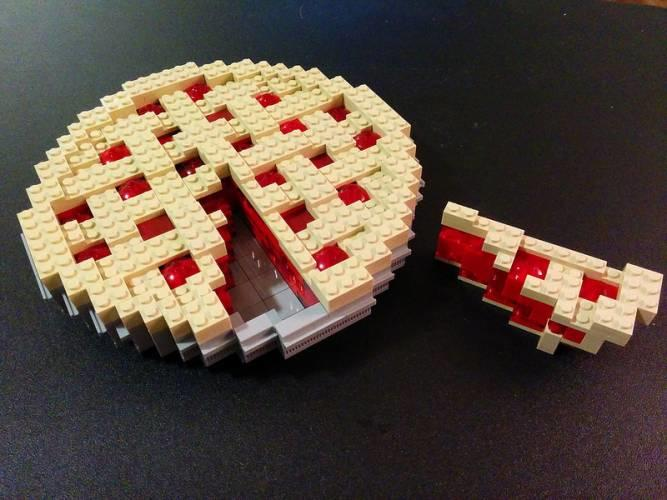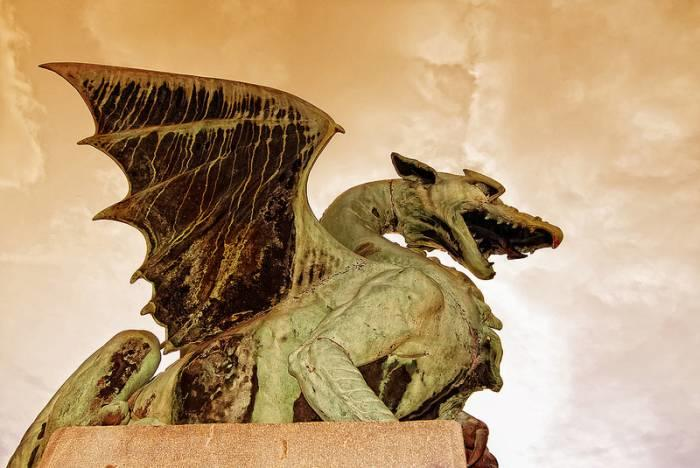The Encyclopaedia Britannica describes Mathematics as “the science of structure, order, and relation that has evolved from elemental practices of counting, measuring, and describing the shapes of objects. It deals with logical reasoning and quantitative calculation, and its development has involved an increasing degree of idealization and abstraction of its subject matter.”

“Mathematics, or its different branches, have been instrumental in the cultural development of practical pursuits such as commerce and agriculture. Equally important is the fact that math has been a source of contemplation and scientific inquiry for generations of scholars.”

The subject matter of mathematics has been a major source of anxiety for many Indian students, indeed students across the world. However, it holds within it many surprising facts and puzzles that have far-reaching applications in the world beyond the classroom.

Find your online maths tutor here.

## Pi and its Enduring Legacy

Pi is an escapable part of not only the discipline of mathematics but our everyday life experiences as well.

The formula of Pi, valued at 3.14 (approximately), is used to calculate the volume of a sphere or the ratio of a circle's circumference to its diameter. It has been a source of intrigue and reverence for mathematicians and scientists throughout history!Pi, a mathematical constant that has its own official day, 14th of March! A day to celebrate the fun maths facts of Pi. Source: visualhunt

The use and application of Pi can be dated back to as early as the Babylonian civilization of 2nd millennium BCE. The Welsh mathematician William Outright is the first documented user of the symbol in 1647. However, it only gained popularity in 1748 with the publication of Leonhard Euler’s “Introduction to Analysis of the Infinite.”

The celebrated mathematician, Archimedes, devised a method to accurately determine the value of Pi and published his findings in the essay, 'Measurement of a Circle' in c. 250 BCE. He was able to figure out a way to estimate the perimeters for polygons with twice as many sides, to arrive at the approximate value of 220/71 <Pi <22/7.

“Archimedes’ principles outlived him by two thousand years!”

### The World is Obsessed with Pi!

Still evolving, Pi has provided mathematicians with more mysteries and paradoxes than perhaps the entire gamut of mathematical theories! Its unique properties have endeared it to the scientific community as well as evoked respect towards it.

• Pi is irrational: It is impossible to write it in fraction form.
• Pi is unpredictable: No mathematical model or computers can predict Pi decimals.
• Pi is transcendent: Pi cannot be the solution to any polynomial equation. However, theoretically, Pi has been linked to mathematical constants, such as the Fibonacci sequence and the Golden Ratio.
• Pi is approximate: The value of Pi is an approximation which, ironically, is used to make accurate calculations.
• Pi is everywhere: Pi is widely employed in the fields of geometry, statistics and probability, and even literature and the Egyptian pyramids!

"Did you know that there is a group of individuals calling themselves the 1000-club who can recite the first thousand decimal places of Pi?"

The contributions of Pi are recognized in the celebration of Pi Day on March 14th every year!

## The Illogical World of Logical-Mathematical Paradoxes

There is an innate human desire to seek explanation, predict the future and explore the unknown that has given birth to the mathematical paradoxes and fallacies. These paradoxes reveal the juxtaposition of mathematics against cultural backdrops that have always existed but seldom observed and acknowledged.

“Here are a few gems for you to ponder about.”

Proposed by the Greek philosopher, Zen of Elea, the paradox retells the well-known fable of the hare and the tortoise, in a mathematical language. It suggested that if the turtle got a head start over the famous Trojan War hero, Achilles, the tortoise would always be ahead in the race.

In order to overtake the tortoise, Achilles must first catch up with it. It got tricky, however, every time Achilles caught up with it as there would be another gap created between the two.

Perceptions of space, time, motion and infinity hold the key to explaining this paradox. The Standard Solution to Zeno’s Paradox was formulated with the help of calculus, a branch of mathematics that has become an indispensable tool in scientific inquiries of the modern world.

“Many of the paradoxes of the old world formed the basis for the development of branches of mathematics such as theorems and mathematical exercises to refute these assertions.”

The Paradox is a logical-mathematical hypothesis, based on a visual illusion. And like all illusions, this one too leads us to make an incorrect conclusion.

In fact, teachers often use this optical illusion to help students hone their logical reasoning skills. The illusion uses two sets of rearranged geometric sets to construct a triangle. But there is an additional geometric square in the second set. How is that possible?

There is actually a simple explanation for this paradox. Neither triangle is a "true" triangle. The small empty space is in fact only the result of a small deformation of the perfect triangle with its slightly rounded edges.

“Show off your knowledge of mathematical trivia and paradoxes in class. Earn some brownie points from your math teacher. You may need them for your next unit test!”

As interdisciplinary studies are gaining traction, students can opt for niche roles in engineering and applied sciences without necessarily earning a Mathematics degree. However, when these assertions or paradoxes were first proposed, they became the topics for much debate among scholars of the time!

## Great Mathematical Mysteries of the World

Mathematics is a lot more than multiplication, division, fractions, algebra, geometry or the several other nightmarish functions that are taught to us in school!

“The subject matter of mathematics, in many ways, contains the essence of the world we inhabit.”

Perhaps, the greatest mathematical mystery is the origin of the discipline itself – was mathematics invented or discovered? Endless debates on the creation/ discovery of mathematics, impossible or unsolved equations, a fascination with certain numbers, unusual formulas, and so on have all contributed to creating a sort of reverence towards the subject. Many mathematical concepts and theories are based or have their origins in religious and mythological beliefs.

India’s contributions to the world of mathematics have been immeasurable. The ancient wisdom contained in the Vedic texts and other scholarly texts of the Early Historic and Early Medieval periods in the Subcontinent contain irrefutable evidence for this.

India can be credited with forming the premise for many mathematical formulas and concepts like the decimal system, numeral system, Zero, calculus, solutions to quadratic equations and a few others.

“The documentary, “The Great Math Mystery” (2015) is a good starting point if you are looking to trace the philosophical debates about the universality and effectiveness of this great discipline.”

### The Big 7

Mathematician D.Hilbert drew up a list of 23 unsolved mathematical problems in 1900 to start the quest for solving the ‘unsolvables’. A century later, in 2000, mathematicians were able to solve the majority of the 23. However, 7 still remain unsolved.

Here are the 7 Millennium Problems.

• The Riemann hypothesis
• The Hodge conjecture
• The Birch and Swinnerton-Dyer conjecture
• The Navier-Stoke equations
• The Yang-Mills Equations
• P versus NP
• Poincaré conjecture

To support research into these scientific mysteries, an American billionaire, L. Clay, set up the CMI (Clay Mathematics Institute) in 1999. He announced a reward of £1 million to anyone who took up the challenge and succeeded at solving any one of these ultimate mathematical problems!

“Very few mathematicians in the world have the opportunity to work on these equations and conjectures. Work harder on your math and ace those tests at school and university.”

### Numbers We All Love!

“Pi tops this list of numbers. No surprises there!”

Some of our other beloved and most recognizable numbers include:

• The Golden Ratio (Phi): Believed to be present in all things in the universe by mathematicians, the Golden Ratio or Divine Proportion is obtained by dividing a line into two parts so that the longer part divided by the smaller part is also equal to the whole length divided by the longer part.
• Drake’s Mysterious Equation: Developed to determine the number of "possible" intelligent civilizations in our galaxy, the equation is yet to be fully deciphered.
• Seven (‘7’): 7 wonders of the world…7 colours of the rainbow…to this day, no scientist has come up with a real explanation for the strong presence of the number 7 in mathematics.

“Is 7 your lucky number? You might want to check with your family astrologer!”

## Using Math to Uncover GOT’s Lead Character

Can math get even more mysterious? And what could everyone’s favourite Game of Thrones have anything to do with the subject most of us love to hate? Seemingly, there is no connection between the two. That was before two math teachers decided to take their curiosity quotient to several levels higher!

Math teachers, A. Beveridge and J. Shan, of MacalesterCollege, wondered if it would be possible to figure out the main character of GOT using a mathematical formula. Their curiosity led them to employ a new science that is based on the mathematical formula of graphs.

### The Networks Theory

Developed in the United States in 2007, the Networks Theory deals with the relationships, connections and interconnections between things.

“Bet, this one definitely got you to sit up and take notice!”I'm starting a petition to include GOT maths games in the maths curriculum across schools nationwide! Who’s with me? Source: visualhunt

They used the theory of graphs and studied the network of information flow between the different characters in the story. They discovered 107 different protagonists (called "vertices") connected by 353 connections (called "edges"). Several measures of centrality were studies in order to model the graph.

AND THE WINNER IS…We won’t reveal it just yet, not here, at least!

“Mathematics is omnipresent.”

From the narrative above, we see how mathematics is embedded in cultures. Discussions around popular culture can take place in class to make math learning more engaging and interactive. Maths games, logic games and magic calculations... there are so many play-and-learn activities out there for you to enjoy!

Come, fall in love with Mathematics!

Need a Maths teacher?5.00/5 - 1 vote(s)Loading...